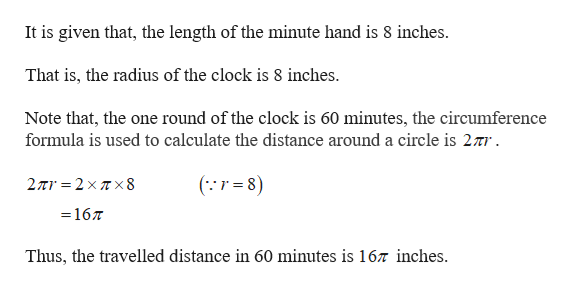# How far does the tip of the minute hand of a clock move in 25 minutes if the hand is 8 inches long?

Question
28 views

How far does the tip of the minute hand of a clock move in 25 minutes if the hand is 8 inches long?

check_circle

Step 1help_outlineImage TranscriptioncloseIt is given that, the length of the minute hand is 8 inches That is, the radius of the clock is 8 inches. Note that, the one round of the clock is 60 minutes, the circumference formula is used to calculate the distance around a circle is 27r (r8) 2π'2xπx8 =167 Thus, the travelled distance in 60 minutes is 167 inches. fullscreen

### Want to see the full answer?

See Solution

#### Want to see this answer and more?

Solutions are written by subject experts who are available 24/7. Questions are typically answered within 1 hour.*

See Solution
*Response times may vary by subject and question.
Tagged in

### Other